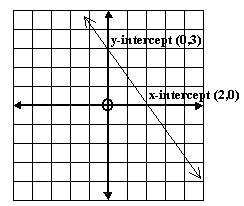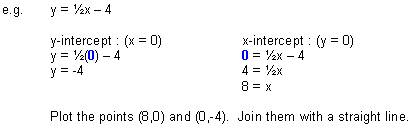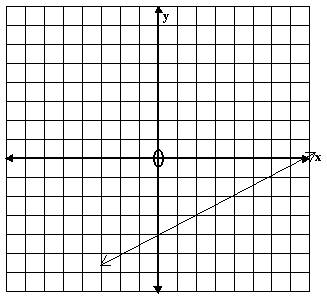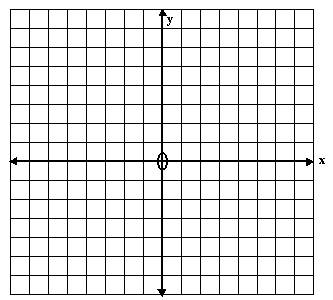# Plotting Straight Line Graphs using Intercept Method Math Review

math tutorials > plotting straight line graphs using intercept method tutorial

## To plot a straight line you need only 2 points

The x-intercept is the point where the graph cuts the x-axis (x,0).  The y-intercept is the point where the graph cuts the y-axis (0,y).1. To calculate the y-intercept, put x = 0.
2. To calculate the x-intercept, put y = 0.
3. Plot the 2 intercepts and join them with a straight line.

## Example## Graph intercept problems for you to try

1)  x + y = 7

2)  y = -x + 2

3)  2y + 3x = 6